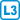## Nash-Wiliams theorem

Show that the edges of each planar graph can be oriented so that each vertex has an outdegree at most 3.

• #### Hint

Choose any orientation and incrementally reduce the outdegrees of the selected vertices by switching the orientation along cleverly selected paths.

• #### Solution

Suppose that in some orientation of a given graph $$G$$ the vertex $$u$$ has an outdegree at least four.

Let us denote by $$S$$ the set of vertices to which exist an oriented path from $$u$$. It holds that the subgraph $$G [S]$$ of the graph $$G$$ induced by the set $$S$$ is also a subgraph $$G$$ induced by the edges that emanate from the vertices $$S$$. Because $$G$$ is planar, $$G [S]$$ is also planar. It follows from Euler’s formula that $$G [S]$$ can have at most $$3 | S | -6$$ edges. Therefore, in $$G [S]$$ necessarily lies the vertex $$v$$, whose outdegree is at most 2 – if such a vertex does not exist, $$G [S]$$ would have at least $$3 | S |$$ edges.

By switching the edges on the path from $$u$$ to $$v$$, we obtain a graph in which the outdegree of $$u$$ is reduced, and the outdegree of $$v$$ does not exceeded three. The outputdegrees of the other vertices remain unchanged.

By repeating the above procedure, we eliminate all vertices whose outdegree is at least four.